## Band Structure p.6

Our aim here is to solve Schrödinger's equation for the "free lattice" using our grid, and to compare the results to the exact analytical solution developed on the previous page. Note that there are at least two things that can go wrong with computer (a.k.a. "numerical") solutions to problems:
1. Limited numerical precision of the computer may limit the accuracy of the result. Of necessity computers can only store a finite number of a number's digits. So, for example, a computer may multiply a 15-digit number by another 15-digit number and be forced to round the result to just 15-digits (plus exponent)...a very small fractional error is made in the process. It is quite possible for the calculation to amplify this small error as many millions of operations are performed. The resulting answer may be far from the correct answer. In our approach to this problem, we approximate derivatives by differences. If the numbers we subtract are very close (say they share the same first 15 digits), subtraction may produce 0, or maybe only one significant digit in the result. Thus if we make h too small we are sure to have problems. (However, probably the first problem we'd run into with smaller h would be very long computer calculation times, as when the griding gets finer, the matrices get bigger and many more operations are required to find the eigenvalues.)

2. The computer approximation may fail to capture the physical problem, so that error results, even if the calculations are done perfectly. For example, with the griding described previously there are just four grid points along the x axis inside the cell. Describing a wave with just four points is going to be very crude. Thus we are led to using finer grids until the problems discussed above become obvious.

Of course, in addition to the above errors, numerical checks are also a good to find blunders, like mistyping the sequence of operations the computer is to perform.

In this particular problem we can actually check all of the above possibilities, because the grid-approximation can itself be exactly solved with pen and paper. Thus we can check if the computer is accurately solving the approximate equations, by seeing how well the exact and computer versions of the approximation agree. We can check if the approximation is similar to reality by comparing the exact solution to the approximate problem to the exact solution to the exact problem.

Ok, here are the numerical results for h=1/8 along our standard sections (computer results are in black):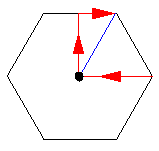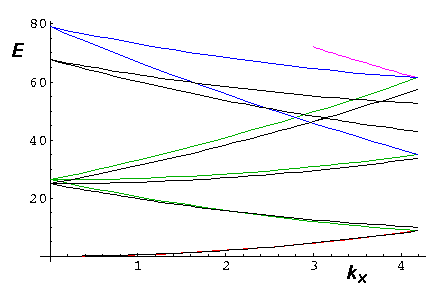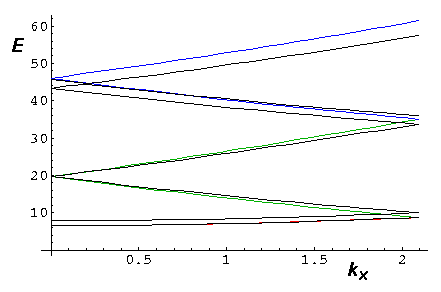First notice that in the region in which we're interested in results (E<25) the calculation closely approximates the exact results. However, at higher energy, the bands are badly off (see, for example, the blue bands in the first plot). Notice that some of the details (e.g., symmetry required degeneracies) are off even at low energy. For example, in the lowest energy band in the zone-boundary plot, the bands should be degenerate, but that are not exactly degenerate in our computational results. Let's see if the exact solution to the approximation will illuminate these differences.

Finding exact solution to the approximate problem is easy. It turns out that the uk that solves the exact problem:

uk=exp(iG·r)

also is the exact eigenvector to the approximate problem. (Yes, this eigenvector does not depend on k; for different bands use different G.) Recall first that this function is periodic, so we don't need to worry about "which" #2 grid point we're talking about. So consider, for example, our finite difference approximation for the derivative w.r.t. x: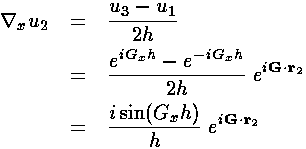The main point here is that action of the derivative matrix on u2 produces a value proportional to u2. Similarly for the other grid points; our candidate u is an eigenvector of the derivative matrix.

For the y derivative and Laplacian, it's the same story: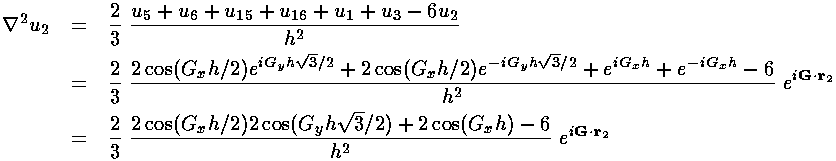Thus our candidate is shown to be an eigenvector of all of the pieces of our matrix, and hence the entire matrix. The eigenenergy is:The main upshot of this is that if h is so small that we can neglect it (and the higher order terms also present), our grided problem's eigenenergies and eigenvectors are identical to the exact solution. Essentially what is required for the second term to be "small" is that Gh be small...a condition not well satisfied for the blue (second-nearest neighbor) solutions. If we decrease h to 1/16 we get much better agreement:So we've now solved the easy problem three ways. We will have but one option when V isn't zero: the numerical eigenproblem for a large matrix. We restrict ourselves to low E where h=1/8 should be good enough.